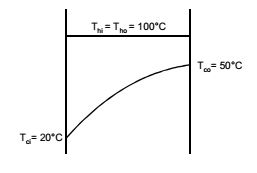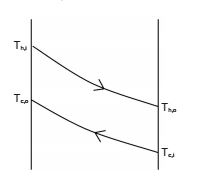## Heat Transfer Miscellaneous

#### Heat-Transfer

1. In a parallel flow heat exchanger operating under steady state, the heat capacity rates (product of specific heat at constant pressure and mass flow rate) of the hot and cold fluid are equal. The hot fluid, flowing at 1 kg/s with cp = 4 kJ/kgK, enters the heat exchanger at 102°C while the cold fluid has an inlet temperature of 15°C. The overall heat transfer coefficient for the heat exchanger is estimated to be 1 kW/m2K and the corresponding heat transfer surface area is 5 m2. Neglect heat transfer between the heat exchanger and the ambient. The heat exchanger is characterized by the following relation: 2ε = 1 – expt(–2NTU). The exit temperature (in °C) for the cold fluid is

1. th1 = 102 °C
tc2 = 15 °C

 NTU = UA = 1 × 103 × 5 Cmin 1 × 4 × 103

 ε = 1 - exp(-2NTU) 2

 = 1 - exp(-2.5) = 0.458 2

 ∴ 0.458 = tc1 - tc2 = tc1 - 15 th1 - th2 102 - 15

or tc1 = 0.458 × (102 – 15) + 15 = 54.846° C ≈ 55°C
Alternately
C = Mc Cc = MhCh = 1 × 4 kJ/kg K
 ∴ C = Mc Cc = 1 MhCh

 NTU = U.A. = 1 × 5 = 5 Cmin 4 4

From the relation
 ε = 1 - e-( 2 × 5 / 4 ) = 0.46 2

 Now , ε = tc2 - 15 = 0.46 102 - 15

∴ 0.46 = tc2
or tc2 = 54.9

##### Correct Option: B

th1 = 102 °C
tc2 = 15 °C

 NTU = UA = 1 × 103 × 5 Cmin 1 × 4 × 103

 ε = 1 - exp(-2NTU) 2

 = 1 - exp(-2.5) = 0.458 2

 ∴ 0.458 = tc1 - tc2 = tc1 - 15 th1 - th2 102 - 15

or tc1 = 0.458 × (102 – 15) + 15 = 54.846° C ≈ 55°C
Alternately
C = Mc Cc = MhCh = 1 × 4 kJ/kg K
 ∴ C = Mc Cc = 1 MhCh

 NTU = U.A. = 1 × 5 = 5 Cmin 4 4

From the relation
 ε = 1 - e-( 2 × 5 / 4 ) = 0.46 2

 Now , ε = tc2 - 15 = 0.46 102 - 15

∴ 0.46 = tc2
or tc2 = 54.9

1. Hot and cold fluids enter a parallel flow double t ube heat exchanger at 100 °C and 15 °C, respectively. The heat capacity rates of hot and cold fluids are Ch = 2000 W/K and Cc = 1200 W/ K, respectively. If the outlet temperature of the cold fluid is 45 °C, the log mean temperature difference (LMTD) of the heat exchanger is _____ K (round off to two decimal places).

1. Hot fluid: (ṁ Cp)h = 2000 W/K
Thi = 100 °C
Cold fluid: (ṁ Cp)c = 1200 W/K
Tci = 15 °C, Tco = 45 °C
(ṁ Cp)h (Thi - Tho) = (ṁ Cp)c (Tco - Tci)
⇒ 2000 × (100 - Tho) = 1200 (45 - 15)
⇒ Tho = 82 °C
(∆Ti) = Thi - Tci = 100 - 15 = 85 °C
(∆To) = Tho - Tco = 82 - 45 = 37 °C

 (LMTD)Parallel = 85° - 37° = 57.71 °C or K ln8537

##### Correct Option: D

Hot fluid: (ṁ Cp)h = 2000 W/K
Thi = 100 °C
Cold fluid: (ṁ Cp)c = 1200 W/K
Tci = 15 °C, Tco = 45 °C
(ṁ Cp)h (Thi - Tho) = (ṁ Cp)c (Tco - Tci)
⇒ 2000 × (100 - Tho) = 1200 (45 - 15)
⇒ Tho = 82 °C
(∆Ti) = Thi - Tci = 100 - 15 = 85 °C
(∆To) = Tho - Tco = 82 - 45 = 37 °C

 (LMTD)Parallel = 85° - 37° = 57.71 °C or K ln8537

1. Saturated steam at 100°C condenses on the outside of a tube. Cold enters the tube at 20°C and exits at 50°C. The value of the Log Mean Temperature Difference (LMTD) is ______°C.

1.Here Tci → cold fluid which enters the tube (temperature)
Tco → cold fluid which exits at tube (temperature)
θ1 = Thi – Tci = 100 – 20 = 80 °C
θ2 = Tho – Tco = 100 – 50 = 50 °C

 θim = θ1 - θ2 ln θ1 θ2

 θim = 80° - 50° = 63.83 °C ln 80 50

##### Correct Option: BHere Tci → cold fluid which enters the tube (temperature)
Tco → cold fluid which exits at tube (temperature)
θ1 = Thi – Tci = 100 – 20 = 80 °C
θ2 = Tho – Tco = 100 – 50 = 50 °C

 θim = θ1 - θ2 ln θ1 θ2

 θim = 80° - 50° = 63.83 °C ln 80 50

1. For a heat exchanger, ∆Tmax is the maximum temperature difference and ∆Tmin is the minimum temperature difference between the two fluids. LMTD is the log mean temperature difference. Cmin and Cmax are the minimum and the maximum heat capacity rates. The maximum possible heat transfer (Qmax) between the two fluids is

1. The temperature difference is not for a given fluid but across the fluids and maximum heat transfer occurs for Cmin and the temperature difference is equal to (Thi - Tci )

##### Correct Option: B

The temperature difference is not for a given fluid but across the fluids and maximum heat transfer occurs for Cmin and the temperature difference is equal to (Thi - Tci )

1. A double pipe counter flow heat exchanger transfers heat between two water streams. Tube side water at 19 litre/s is heated frim 10°C to 38°C. Shell side water at 25 litre/s is entering at 46°C Assume constant properties of water, density is 1000 kg/m3 and specific heat is 4186 J/kgK. The LMTD (in °C) is ____.

1. Given: ṁh = 25 L/S; Th,i = 46°C, Th,o =?
c = 19 L/S; Tc,i = 10°C, Tc,o = 38°C
ρ = 1000 kg/m33, C = 4186 J/kg.k
Energy balance, ṁc = C(Tc,o – Tc,i) = mn C(Th,i – Th,o)
19 (38 – 10) = 25 (46 – Th,o)
Th,o = 24.72 °CLMTD = θ1 - θ2 ln θ1 θ2

θ1 = Th,i – Tc,o = 46 – 38 = 8 °C
θ2 = Th,o – Tc,i = 24.72 – 10 = 14.72
 LMTD = 8 - 14.72 = 11.0206°C ln 8 14.72

##### Correct Option: A

Given: ṁh = 25 L/S; Th,i = 46°C, Th,o =?
c = 19 L/S; Tc,i = 10°C, Tc,o = 38°C
ρ = 1000 kg/m33, C = 4186 J/kg.k
Energy balance, ṁc = C(Tc,o – Tc,i) = mn C(Th,i – Th,o)
19 (38 – 10) = 25 (46 – Th,o)
Th,o = 24.72 °CLMTD = θ1 - θ2 ln θ1 θ2

θ1 = Th,i – Tc,o = 46 – 38 = 8 °C
θ2 = Th,o – Tc,i = 24.72 – 10 = 14.72
 LMTD = 8 - 14.72 = 11.0206°C ln 8 14.72